# Introduction to Computer Vision with OpenCV

May 25, 2022 • 19 min read### Computer Vision

Computer vision (CV) is a field of artificial intelligence that allows computers to detect and understand the visual world. It is commonly used to derive meaningful information from given images and videos.

With the outstanding rise of the artificial intelligence field, computer vision is becoming more and more prominent by the day. Computer vision opens new paths to machine learning, deep learning, and artificial intelligence in general. Using dedicated computer vision libraries like OpenCV to execute computer vision assignments faster and easier.

### OpenCV

OpenCV is an open-source library that contains plenty of useful functions aimed mainly at computer vision and machine learning. This library can work with multiple interfaces (computer languages) such as Python, C++, Java, and MATLAB.

Our main focus for this tutorial would be an introduction to Computer Vision using OpenCV. For this tutorial, we are going to write our code using Python. If you do not have Python installed on your device, then please download the latest version available from here.

In this tutorial, we will go through some of the basic functionality of OpenCV and demonstrate advanced real-life applications.

#### Installing OpenCV

In this tutorial, we are going to use the pip function in order to install OpenCV. If you do not have pip already installed on your device then please download it from the following link.

To install the OpenCV library copy-paste the following code into your terminal of choice:

`pip install opencv-contrib-python`

Note that this is not your normal OpenCV library as it contains more functions that may come in handy.

#### Importing Necessary Libraries

Before importing the cv2 library, make sure that you have installed OpenCV on your device. You should always have the cv2 library imported when using any of its functions. Note that in some parts of this tutorial you may find the cv import missing, but you should always include it.

`from cv2 import cv`

#### Reading and Viewing an Image

When it comes to OpenCV and computer vision in general a good starting point would be to read given images and videos.

To start with the first basic function we will be reading an image using the OpenCV library.

Using the imread function provided by the cv2 library, you can easily read given images and videos.

`image = cv.imread("Image Path")`

To view or show a given image:

The imshow function takes the name of the window you want to show. In our case we called it “Image”, and it takes the image we want to view as the second parameter.

`cv.imshow("Image", image)`

Note that you should add the waitkey function at the end of your code in order for the imshow function to work. The waitkey function takes only one parameter which is the delay time(in milliseconds) that the image window will remain open before pressing any key.

`cv.waitKey(0)cv.destroyAllWindows()`

The below function divides a given video into single frames(images) and then continuously displays every single frame in a loop. If the “q” letter is pressed then we will break out of the loop and the video will stop.

`video_cap = cv.VideoCapture("Video Path")while True:   isSuccess, frame = video_cap.read()   cv.imshow("Video", frame)if cv.waitKey(20) & 0xFF == ord("q"):       breakvideo_cap.release()cv.destroyAllWindows()`

Make sure to add the cv.waitKey(0) inside your While loop. This allows you to create and then destroy each frame until the frames are finished.

#### Rescaling Images

Using the cv.resize(src, dsize, dst, fx, fy, interpolation) function which is provided in the OpenCV library we can freely resize a given image. The resize function takes an image as its first parameter and the scaling dimension required as its second parameter. The fx is the scaling factor along the horizontal axis and the fy is the scaling along the vertical axis. For the final parameter which is the interpolation attribute, we provide the preferred sampling method. In our case we chose INTER_AREA.

`image = cv.imread("Image Path")scale = 0.5 # default scale valueheight = int(image.shape*scale)width = int(image.shape*scale)dim(width, height)resizedImage = cv.resize(image, dim, interpolation = cv.INTER_AREAcv.imshow("Resized Image",  resizedImage)cv.waitKey(0)cv2.destroyAllWindows()`

Note you can always increase the size of the image by providing a scaling factor greater than one.

### Image Manipulation

In this part, we will mention some major image manipulation techniques that may come in handy.

#### Converting Image to GrayScale

By converting an image into a grayscale format, you are simply removing the Red, Green, and Blue channels from your image. This would decrease the total bit size from 24 bits ( 8-bits for each color ) to 8 (grayScale) bits. This can improve your model’s performance with no noticeable effect at all as some models do not require color identification in order to perform.

`image = cv.imread("Photo Path")cv.imshow("Original Image", image)grayScaleImage = cv.cvtColor(image, cv.COLOR_BGR2GRAY)cv.imshow("Gray Scale Image", grayScaleImage)cv.waitKey(0)`

#### Blurring Images

Blurring an image allows you to reduce the excess noise available. Moreover, blurring an image will reduce computation time.

There are a couple of different blurring algorithms:

#### 1. Average Blurring

The average blurring method averages all the 8 pixels surrounding a given pixel and gives the middle pixel the result as its new value.

`image = cv.imread("Photo Path")cv.imshow("Original Image", image)blur = cv.blur(image, (5,5))cv.imshow("image", blur)`

The blur function takes the image and the specified size of the kernel. Increasing the size value will increase the blurriness of the image.

#### 2. Gaussian Blur

Similar to the average blur but with the addition of having each surrounding pixel having its own weight.

`image = cv.imread("Photo Path")cv.imshow("Original Image", image)gaussianImage = cv.GaussianBlur(image, (5,5), 0)cv.imshow("image", gaussianImage)cv.waitKey(0)`

#### 3. Median Blur

Similar to average blur, median blur finds the median of the surrounding pixels instead of the average.

#### 4. Bilateral Blur

Bilateral blurring does blur the image while still maintaining its edges.

`image = cv.imread("Photo Path")bilateral = cv.bilateralFilter(image, 10, 10, 10)cv.imshow("image", bilateral)cv.waitKey(0)`

### Color Channels

In this part, we will learn how to split an image into the three color channels ( Blue, Green, and Red ) by using the split() function. The split function will split our image into 8-bit images, meaning that the 24-bit image will be split into three 8-bit images. This will result in 3 grayscale images with each image representing the intensity of each of the RGB colors in black and white.

`from cv2 import cv2import numpy as npimage = cv.imread("Photo Path")b,g,r = cv.split(image)cv.imshow("Blue", b)cv.imshow("Green", g)cv.imshow("Red", r)cv.waitKey(0)`
 Blue Image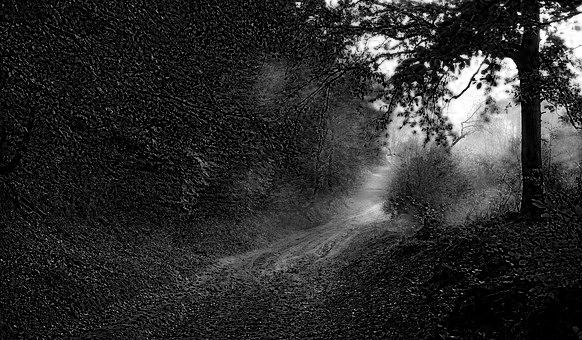Green Image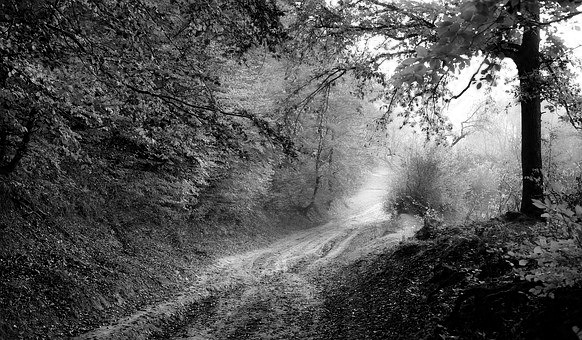Red ImageTo reflect the RGB colors in each of the 8-bit images we are going to merge each image with 2 other blank images. Note that these blank images will have no effect at all on our image, but they will return our image back into a 24-bit image.

The blank image will set the color to black, allowing only the chosen color to be visible.

 Blue Image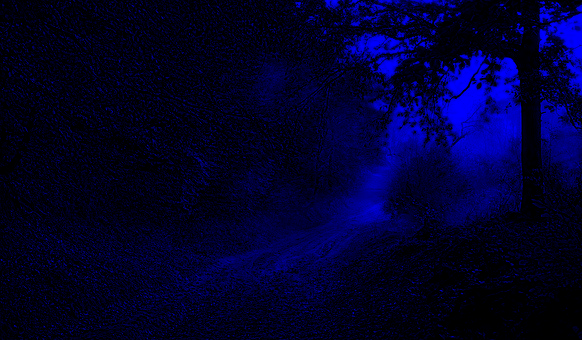Green Image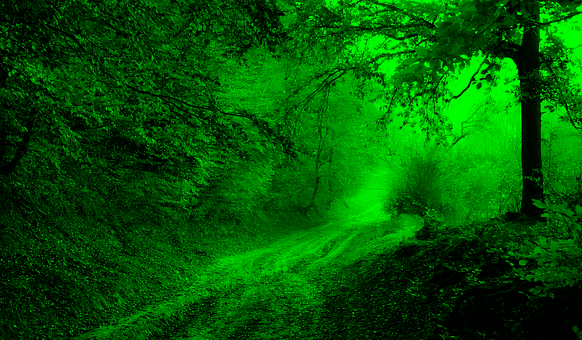Red Image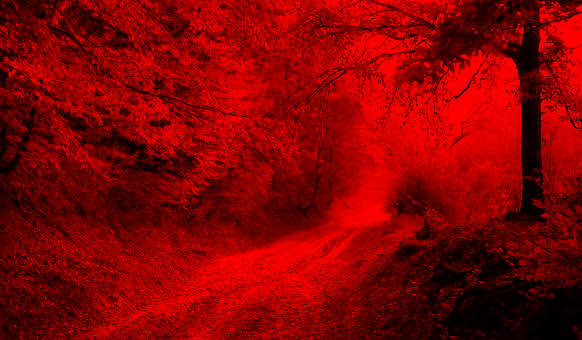### Edge Detection

Extracting the edges of an image is very useful in both computer vision and machine learning fields. As it allows us to detect different objects and shapes, which we can use for later models. We are going to use two different edge detection algorithms on the below image.

#### 1. Sobel Edge Detection

`from cv2 import cvimport numpy as npimage = cv.imread("Photo Path")grayScaleImage = cv.cvtColor(image, cv.COLOR_BGR2GRAY)Sobel_x = cv.sobel(grayScaleImage, cv.CV_64F, 0, 1)Sobel_y = cv.sobel(grayScaleImage, cv.CV_64F, 1, 0)cv.imshow("Sobel_x image", Sobel_x)cv.imshow("Sobel_y image", Sobel_y)Sobel = cv.bitwise_or(Sobel_x, Sobel_y)cv.imshow("Sobel image", Sobel)cv.waitKey(0)`

#### 2. Canny Edge Detection

The Canny function runs a more advanced algorithm than Sobel. The first argument of the canny function is the source image, while the second and third arguments are the threshold values which are the min and max values. These two arguments are used to detect the edges. If a value is below the 100 threshold mark then it is not considered an edge. Edges that fall between the 100 and 200 mark are classified depending on their connectivity. Finally, all values above 200 are sure to be edges.

`from cv2 import cvimport numpy as npimage = cv.imread("Photo Path")grayScaleImage = cv.cvtColor(image, cv.COLOR_BGR2GRAY)Canny = cv.Canny(grayScaleImage, 100, 200)cv.imshow("Canny image", Canny)cv.waitKey(0)`

Note that the Canny algorithm will provide a cleaner image than the normal Sobel function.

### Circle Detection

For our first real-life implementation, we are going to detect all the circles in the below image.

To start with, we will be using the Hough Circles function in order to detect the black circle. To find the original code by Adrian.

`# import the necessary packagesfrom cv2 import cv2import numpy as npimport argparse# construct the argument parser and parse the argumentsap = argparse.ArgumentParser()ap.add_argument("-C", "-/Path", required = False, help = "Path to the image")# load the image, clone it for output, and then convert it to grayscaleimage = cv2.imread("Path")output = image.copy()gray = cv2.cvtColor(image, cv2.COLOR_BGR2GRAY)# detect circles in the imagecircles = cv2.HoughCircles(gray, cv2.HOUGH_GRADIENT, 1, 1)# ensure at least some circles were foundif circles is not None:# convert the (x, y) coordinates and radius of the circles to integerscircles = np.round(circles[0, :]).astype("int")# loop over the (x, y) coordinates and radius of the circlesfor (x, y, r) in circles:# draw the circle in the output image, then draw a rectangle# corresponding to the center of the circlecv2.circle(output, (x, y), r, (0, 255, 0), 4)cv2.rectangle(output, (x - 5, y - 5), (x + 5, y + 5), (0, 128, 255), -1)# show the output imagecv2.imshow("output", output)cv2.waitKey(0)cv2.destroyAllWindows()`

As shown above, our code detects any circular-shaped object and draws a green line around the circumference and a small orange square in the center of the found circle.

For us to detect the circles in the below image the value of the second parameter in the HoughCircles function must be 1.1.

 Original Circles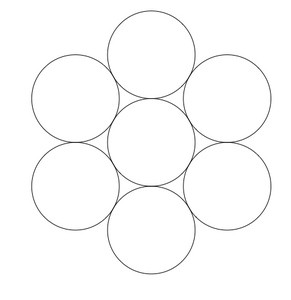Detected Circles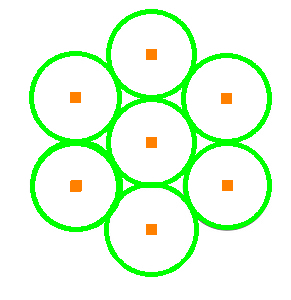### ArUco Marker Detection

In this part, we learn how to detect and decode a given ArUco marker. Let’s start by explaining what an ArUco marker is first.

Similar to QR codes, ArUco markers are fiducial markers that can be placed on multiple surfaces. ArUco markers can have data encoded into them, moreover, they can also provide accurate localization.

ArUco codes are made of a black background and white squares. They do come in different sizes depending on the amount of data you want to store. You can find the original code by Sunita here.

The below code allows you to generate an ArUco marker

`dictionary = cv2.aruco.Dictionary_get(cv2.aruco.DICT_4X4_50)`

Using the Dictionary_get() function you can choose the matrix size of the marker required. In our case, we chose a 4 by 4 marker with up to 50 values that can be encoded on it.

`marker = np.zeros((200, 200), dtype=np.uint8)marker = cv2.aruco.drawMarker(dictionary, 5, 200, marker, 1)cv2.imshow("marker image", marker)`

The first drawMarker function parameter takes the chosen dictionary of the marker. The second parameter will be the value you want to encode into your marker, we chose a value of 5. The third input will be the matrix size which is 200x200 bits. For the fourth parameter, we will pass the plain image from the previous line. The last parameter will border our matrix with a single black square from all sides. It's always preferred to have at least a 1-bit black border to easily detect the matrix.

The below code surrounds the ArUco marker with a white border

`marker_with_whiteborder = cv2.copyMakeBorder(marker, 20, 20, 20, 20, cv2.BORDER_CONSTANT, value=[255, 255, 0])`

Note that for the detectMarkers() function to detect the given ArUco marker, a surrounding white border may be necessary for the algorithm to differentiate between the marker's black borders and the image’s background.

The below code allows you to detect and decode ArUco markers

`parameters =  cv2.aruco.DetectorParameters_create()markerCorners, markerIds, rejectedCandidates =cv2.aruco.detectMarkers(marker_with_whiteborder, dictionary, parameters=parameters)print("markers ID", markerIds)`

You can also print the encoded markers ID into the right bottom corner of the original image by using:

`ID = str(markerIds)cv2.putText(img=marker_with_whiteborder, text=ID, org=(100, 100),fontFace=cv2.FONT_HERSHEY_SIMPLEX , fontScale=0.5, color=(125,125,125), thickness=1)cv2.imshow("output", marker_with_whiteborder)cv2.waitKey(0)`

The putText function allows you to print a given text onto an image. This function can take the image, text location, font, font scale, color, and thickness as inputs.

### Conclusion

To sum it up, we learned some of the basic computer vision functions, including reading images and videos, rescaling images, multiple blurring techniques, and how to work with different color channels. Then we moved to more advanced topics like edge detection, circle detection, and finally ArUco markers detection and decoding.

Whether you are studying computer vision to advance your career, or for fun, learning more about the basic functions provided by the OpenCV library and other general computer vision functions can be extremely valuable.

SabrePC can help find a solution that fits your workload. If you have any questions or want to suggest some other topics for us to focus on, please feel free to contact us.

computer vision

deep learning

opencv

how to

tutorial